# Rotations of order 4 and reflections

The remaining patterns are numbered 211, which implies that one more further question needs to the answered.

The first group are examples of patterns with equilateral triangles. An example of two of these are: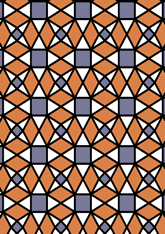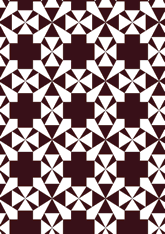The number in this group is 22, which is 10% of the total. Another question is needed - for this see page.
The second group are examples of patterns with squares. An example of two of these are: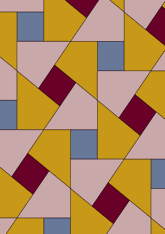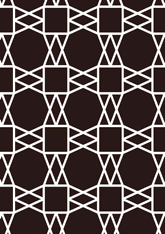The number in this group is 63, which is 29% of the total. Another question is needed - for this see page.
The third group are examples of patterns with diamonds. An example of two of these are: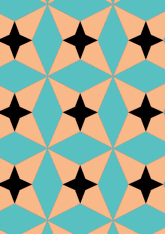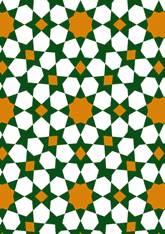The number in this group is 41, which is 19% of the total. Another question is needed - for this see page.
The last group are examples of patterns which are none of the above. An example of two of these are: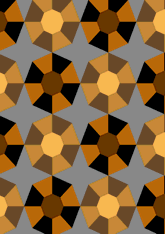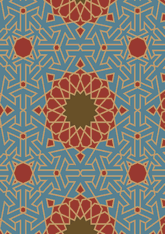The number in this group is 85, which is 40% of the total. Another question is needed - for this see page.

v53

Tiling Search was created by Brian Wichmann, and is maintained by MIT Libraries, through support from the Aga Khan Documentation Center.

Also supported by Performant Software Solutions LLC.